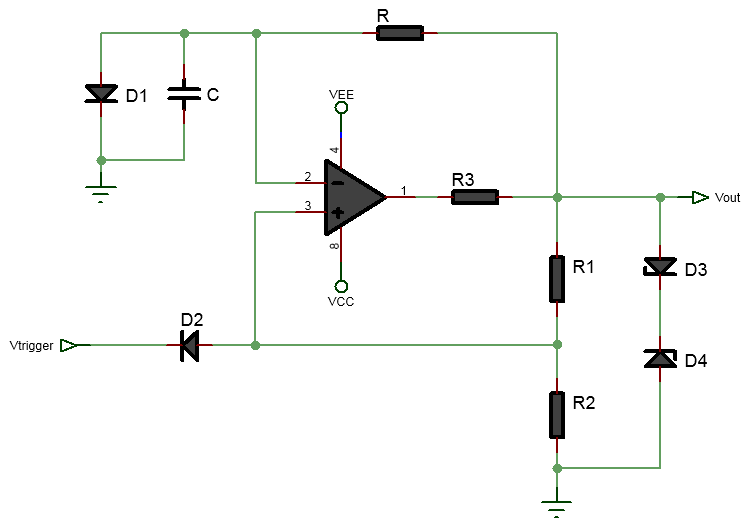# Op-Amp Monstable Multivibrator Calculator

Output:The above online op-amp based monostable multivibrator calculator is a tool designed to assist engineers and hobbyists in designing monostable multivibrator circuits using operational amplifiers (op-amps). The calculator provides a simple and user-friendly interface that allows users to input various parameters and obtain the desired output values for their monostable multivibrator circuit.

The calculator typically includes the following input fields: Trigger Pulse Width (T): This is the desired duration of the output pulse generated by the monostable multivibrator circuit. Users can input the desired pulse width in seconds, milliseconds, or microseconds. Capacitor Value (C): This is the value of the capacitor used in the timing circuit of the monostable multivibrator. Users can input the desired capacitance value in farads, microfarads, nanofarads, or picofarads. Based on these input parameters, the calculator then computes and displays the Timing Resistor (R).

This is the calculated using the following formula and assumptions:

$$R = \frac{T}{0.69RC}$$

References: